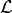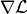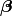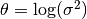# Supplement¶

## Bayesian Linear Regression Model¶

The unnormalized posterior distribution was given for a Bayesian Linear Regression Model in the tutorial. Additional forms of that posterior are given in the following section.

### Log-Transformed Distribution and Gradient¶

Letdenote the logarithm of a density of interest up to a normalizing constant, andits gradient. Then, the following are obtained for the regression example parametersand, for samplers, like NUTS, that can utilize both.